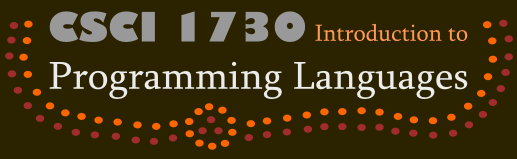### Type Inference

Complete this assignment with the same team you worked with for Types (Written). You and your partner must each understand the answers to all the problems, so don't just split up the work.

#### Part I: Parsing the Language

Write a parser for this language:

``` <expr> ::= <num>
| true
| false
| {+ <expr> <expr>}
| {- <expr> <expr>}
| {* <expr> <expr>}
| {iszero <expr>}
| {bif <expr> <expr> <expr>}

| <id>
| {with {<id> <expr>} <expr>}
| {rec {<id> <expr>} <expr>}
| {fun {<id>} <expr>}
| {<expr> <expr>}

| tempty
| {tcons <expr> <expr>}
| {tempty? <expr>}
| {tfirst <expr>}
| {trest <expr>}
```

The support code specifies the abstract syntax that your parser should return. Your parse function must have the name and contract

`parse :: S-exp -> Expr`
You may assume that the s-expressions supplied to `parse` are valid and closed.

#### Part II: Constraint Generation

Write a function called `generate-constraints` that accepts an expression and returns a list of constraints. You have the freedom to determine the precise contract of this function, including the representation of your constraints. However, we strongly recommend that you use the `Type` data structures as defined in the support code as part of your representation of constraints. A few things to note:

• List operations are polymorphic; that is, you can create lists of numbers or booleans.

• During constraint generation, you will need to generate fresh identifiers. The function `gensym` returns a unique symbol each time it is applied. (`gensym` accepts an optional symbol as an argument; the result of `(gensym 'x)` is then a unique symbol that "looks like " `'x`.)

#### Part III: Unification

Implement the unification algorithm from the lecture notes. Call the function `unify`. The `unify` function should accept a list of constraints and return a substitution. However, `unify` should signal an error if two types cannot be unified or if the occurs check fails. Again, the precise contract of `unify` is up to you to define.

#### Part IV: Inferring Types

To infer the type of a program, parse it, generate constraints, and unify the constraints to get a substitution. From the substitution, you can determine the type of the program.

In particular, define the function

`infer-type :: Expr -> Type`

#### Part V: Testing and TestFest

`infer-type` returns a `Type`, so you will need to test types for equality. This can be tricky due to type variables, particularly if `generate-constraints` calls `(gensym)` to generate unique type variables. The support code defines a function ```((type=? t1) t2)``` that returns `true` if `t1` and `t2` are equal, modulo renaming of variables. We've included a few examples that show you how to use `type=?` with `test/pred` and `test/exn`. You are free to modify this function as you see fit or to not use it at all.

You should write unit tests for all functions that you write. However, for the purpose of TestFest, ensure that your test suites just use ```parse :: S-exp -> Expr``` and `infer-type :: Expr -> Type`. If you chose to use the `type=?` function we have provided, include it with your test suite.

For fun, try to write a program in this language for which your algorithm infers the type

`(t-fun (t-var 'a) (t-var 'b))`

#### What Not To Do

You do not need to implement an interpreter for this language.

You do not need to implement let-based polymorphism.

#### Handin

A single member of your team should handin the assignment. From the directory containing the files for the assignment you wish to hand in, execute

`/course/cs173/bin/cs173handin typeinf-prog`